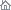Home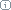About Us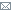ContactBlogWhat's NewProductsBuy NowDownloadsForumGeneXproTools Online Guide Learn how to use the 5 modeling platforms of GeneXproTools with the Online GuideLast update: February 19, 2014Built-in Mathematical Functions

Below are listed all the 279 built-in mathematical functions available in GeneXproTools, including their representation in Karva Notation (shown in red) and their mathematical definition (for more details about their implementation, see the built-in grammars of GeneXproTools):

Basic arithmetic functions:

• Subtraction (-): (x-y)
• Multiplication (*): (x*y)
• Division (/): (x/y)
• Subtraction with 3 inputs (Sub3): (x-y-z)
• Multiplication with 3 inputs (Mul3): (x*y*z)
• Division with 3 inputs (Div3): (x/y/z)
• Subtraction with 4 inputs (Sub4): (a-b-c-d)
• Multiplication with 4 inputs (Mul4): (a*b*c*d)
• Division with 4 inputs (Div4): (a/b/c/d)

Logarithmic functions:

• Natural logarithm (Ln): ln(x)
• Logarithm of base 10 (Log): log(x)
• Logarithm(x,y) (Log2): log(x,y)

Exponential and power functions:

• Square root (Sqrt): sqrt(x)
• Exponential (Exp): exp(x)
• Power (Pow): xy
• 10x (Pow10): 10x
• x2 (X2): x2
• x3 (X3): x3
• x4 (X4): x4
• x5 (X5): x5
• Cube root (3Rt): x(1/3)
• Quartic root (4Rt): x(1/4)
• Quintic root (5Rt): x(1/5)
• Logistic(x) (Logi): logistic(x)
• Logistic(x,y) (Logi2): logistic(x,y)
• Logistic(x,y,z) (Logi3): logistic(x,y,z)
• Logistic(a,b,c,d) (Logi4): logistic(a,b,c,d)
• Gaussian(x) (Gau): gaussian(x)
• Gaussian(x,y) (Gau2): gaussian(x,y)
• Gaussian(x,y,z) (Gau3): gaussian(x,y,z)
• Gaussian(a,b,c,d) (Gau4): gaussian(a,b,c,d)

Rounding and other simple functions:

• Floor (Floor): floor(x)
• Ceiling (Ceil): ceil(x)
• Floating-point remainder (Mod): mod(x,y)
• Absolute value (Abs): abs(x)
• Inverse (Inv): 1/x
• Negation (Neg): -x
• Complement (NOT): (1-x)
• No operation (Nop): x

Minimum, maximum, and average functions:

• Minimum of 2 inputs (Min2): min(x,y)
• Minimum of 3 inputs (Min3): min(x,y,z)
• Minimum of 4 inputs (Min4): min(a,b,c,d)
• Maximum of 2 inputs (Max2): max(x,y)
• Maximum of 3 inputs (Max3): max(x,y,z)
• Maximum of 4 inputs (Max4): max(a,b,c,d)
• Average of 2 inputs (Avg2): avg(x,y)
• Average of 3 inputs (Avg3): avg(x,y,z)
• Average of 4 inputs (Avg4): avg(a,b,c,d)

Constant functions:

• Constant zero function (Zero): 0(x) = 0
• Constant one function (One): 1(x) = 1
• 0(x,y) (Zero2): 0(x,y) = 0
• 1(x,y) (One2): 1(x,y) = 1
• Number p (Pi): Pi(x) = 3.141592653589793
• Euler's number (E): E(x) = 2.718281828459045

Trigonometric functions (x is in radians):

• Sine (Sin): sin(x)
• Cosine (Cos): cos(x)
• Tangent (Tan): tan(x)
• Cosecant (Csc): csc(x)
• Secant (Sec): sec(x)
• Cotangent (Cot): cot(x)

Inverse trigonometric functions:

• Arcsine (Asin): arcsin(x)
• Arccosine (Acos): arccos(x)
• Arctangent (Atan): arctan(x)
• Arccosecant (Acsc): arccsc(x)
• Arcsecant (Asec): arcsec(x)
• Arccotangent (Acot): arccot(x)

Hyperbolic functions:

• Hyperbolic sine (Sinh): sinh(x)
• Hyperbolic cosine (Cosh): cosh(x)
• Hyperbolic tangent (Tanh): tanh(x)
• Hyperbolic cosecant (Csch): csch(x)
• Hyperbolic secant (Sech): sech(x)
• Hyperbolic cotangent (Coth): coth(x)

Inverse hyperbolic functions:

• Inverse hyperbolic sine (Asinh): arcsinh(x)
• Inverse hyperbolic cosine (Acosh): arccosh(x)
• Inverse hyperbolic tangent (Atanh): arctanh(x)
• Inverse hyperbolic cosecant (Acsch): arccsch(x)
• Inverse hyperbolic secant (Asech): arcsech(x)
• Inverse hyperbolic cotangent (Acoth): arccoth(x)

Comparison 0/1 functions of two arguments (OR series):

• OR1 (OR1): if x < 0 OR y < 0, then 1, else 0
• OR2 (OR2): if x >= 0 OR y >= 0, then 1, else 0
• OR3 (OR3): if x <= 0 OR y <= 0, then 1, else 0
• OR4 (OR4): if x < 1 OR y < 1, then 1, else 0
• OR5 (OR5): if x >= 1 OR y >= 1, then 1, else 0
• OR6 (OR6): if x <= 1 OR y <= 1, then 1, else 0

Comparison 0/1 functions of two arguments (AND series):

• AND1 (AND1): if x < 0 AND y < 0, then 1, else 0
• AND2 (AND2): if x >= 0 AND y >= 0, then 1, else 0
• AND3 (AND3): if x <= 0 AND y <= 0, then 1, else 0
• AND4 (AND4): if x < 1 AND y < 1, then 1, else 0
• AND5 (AND5): if x >= 1 AND y >= 1, then 1, else 0
• AND6 (AND6): if x <= 1 AND y <= 1, then 1, else 0

Comparison IF THEN ELSE functions of two arguments (series A):

• Less Than with 2 inputs (A) (LT2A): if x < y, then x, else y
• Greater Than with 2 inputs (A) (GT2A): if x > y, then x, else y
• Less Or Equal To with 2 inputs (A) (LOE2A): if x <= y, then x, else y
• Greater Or Equal To with 2 inputs (A) (GOE2A): if x >= y, then x, else y
• Equal To with 2 inputs (A) (ET2A): if x = y, then x, else y
• Not Equal To with 2 inputs (A) (NET2A): if x != y, then x, else y

Comparison 0/1 IF THEN ELSE functions of two arguments (series B):

• Less Than with 2 inputs (B) (LT2B): if x < y, then 1, else 0
• Greater Than with 2 inputs (B) (GT2B): if x > y, then 1, else 0
• Less Or Equal To with 2 inputs (B) (LOE2B): if x <= y, then 1, else 0
• Greater Or Equal To with 2 inputs (B) (GOE2B): if x >= y, then 1, else 0
• Equal To with 2 inputs (B) (ET2B): if x = y, then 1, else 0
• Not Equal To with 2 inputs (B) (NET2B): if x != y, then 1, else 0

Comparison IF THEN ELSE functions of two arguments (series C):

• Less Than with 2 inputs (C) (LT2C): if x < y, then (x+y), else (x-y)
• Greater Than with 2 inputs (C) (GT2C): if x > y, then (x+y), else (x-y)
• Less Or Equal To with 2 inputs (C) (LOE2C): if x <= y, then (x+y), else (x-y)
• Greater Or Equal To with 2 inputs (C) (GOE2C): if x >= y, then (x+y), else (x-y)
• Equal To with 2 inputs (C) (ET2C): if x = y, then (x+y), else (x-y)
• Not Equal To with 2 inputs (C) (NET2C): if x != y, then (x+y), else (x-y)

Comparison IF THEN ELSE functions of two arguments (series D):

• Less Than with 2 inputs (D) (LT2D): if x < y, then (x*y), else (x/y)
• Greater Than with 2 inputs (D) (GT2D): if x > y, then (x*y), else (x/y)
• Less Or Equal To with 2 inputs (D) (LOE2D): if x <= y, then (x*y), else (x/y)
• Greater Or Equal To with 2 inputs (D) (GOE2D): if x >= y, then (x*y), else (x/y)
• Equal To with 2 inputs (D) (ET2D): if x = y, then (x*y), else (x/y)
• Not Equal To with 2 inputs (D) (NET2D): if x != y, then (x*y), else (x/y)

Comparison IF THEN ELSE functions of two arguments (series E):

• Less Than with 2 inputs (E) (LT2E): if x < y, then (x+y), else (x*y)
• Greater Than with 2 inputs (E) (GT2E): if x > y, then (x+y), else (x*y)
• Less Or Equal To with 2 inputs (E) (LOE2E): if x <= y, then (x+y), else (x*y)
• Greater Or Equal To with 2 inputs (E) (GOE2E): if x >= y, then (x+y), else (x*y)
• Equal To with 2 inputs (E) (ET2E): if x = y, then (x+y), else (x*y)
• Not Equal To with 2 inputs (E) (NET2E): if x != y, then (x+y), else (x*y)

Comparison IF THEN ELSE functions of two arguments (series F):

• Less Than with 2 inputs (F) (LT2F): if x < y, then (x+y), else sin(x*y)
• Greater Than with 2 inputs (F) (GT2F): if x > y, then (x+y), else sin(x*y)
• Less Or Equal To with 2 inputs (F) (LOE2F): if x <= y, then (x+y), else sin(x*y)
• Greater Or Equal To with 2 inputs (F) (GOE2F): if x >= y, then (x+y), else sin(x*y)
• Equal To with 2 inputs (F) (ET2F): if x = y, then (x+y), else sin(x*y)
• Not Equal To with 2 inputs (F) (NET2F): if x != y, then (x+y), else sin(x*y)

Comparison IF THEN ELSE functions of two arguments (series G):

• Less Than with 2 inputs (G) (LT2G): if x < y, then (x+y), else atan(x*y)
• Greater Than with 2 inputs (G) (GT2G): if x > y, then (x+y), else atan(x*y)
• Less Or Equal To with 2 inputs (G) (LOE2G): if x <= y, then (x+y), else atan(x*y)
• Greater Or Equal To with 2 inputs (G) (GOE2G): if x >= y, then (x+y), else atan(x*y)
• Equal To with 2 inputs (G) (ET2G): if x = y, then (x+y), else atan(x*y)
• Not Equal To with 2 inputs (G) (NET2G): if x != y, then (x+y), else atan(x*y)

Comparison IF THEN ELSE functions of three arguments (series A):

• Less Than with 3 inputs (A) (LT3A): if x < 0, then y, else z
• Greater Than with 3 inputs (A) (GT3A): if x > 0, then y, else z
• Less Or Equal To with 3 inputs (A) (LOE3A): if x <= 0, then y, else z
• Greater Or Equal To with 3 inputs (A) (GOE3A): if x >= 0, then y, else z
• Equal To with 3 inputs (A) (ET3A): if x = 0, then y, else z
• Not Equal To with 3 inputs (A) (NET3A): if x != 0, then y, else z

Comparison IF THEN ELSE functions of three arguments (series B):

• Less Than with 3 inputs (B) (LT3B): if (x+y) < z, then (x+y), else z
• Greater Than with 3 inputs (B) (GT3B): if (x+y) > z, then (x+y), else z
• Less Or Equal To with 3 inputs (B) (LOE3B): if (x+y) <= z, then (x+y), else z
• Greater Or Equal To with 3 inputs (B) (GOE3B): if (x+y) >= z, then (x+y), else z
• Equal To with 3 inputs (B) (ET3B): if (x+y) = z, then (x+y), else z
• Not Equal To with 3 inputs (B) (NET3B): if (x+y) != z, then (x+y), else z

Comparison IF THEN ELSE functions of three arguments (series C):

• Less Than with 3 inputs (C) (LT3C): if (x+y) < z, then (x+y), else (x+z)
• Greater Than with 3 inputs (C) (GT3C): if (x+y) > z, then (x+y), else (x+z)
• Less Or Equal To with 3 inputs (C) (LOE3C): if (x+y) <= z, then (x+y), else (x+z)
• Greater Or Equal To with 3 inputs (C) (GOE3C): if (x+y) >= z, then (x+y), else (x+z)
• Equal To with 3 inputs (C) (ET3C): if (x+y) = z, then (x+y), else (x+z)
• Not Equal To with 3 inputs (C) (NET3C): if (x+y) != z, then (x+y), else (x+z)

Comparison IF THEN ELSE functions of three arguments (series D):

• Less Than with 3 inputs (D) (LT3D): if (x+y) < z, then (x+y), else (x-z)
• Greater Than with 3 inputs (D) (GT3D): if (x+y) > z, then (x+y), else (x-z)
• Less Or Equal To with 3 inputs (D) (LOE3D): if (x+y) <= z, then (x+y), else (x-z)
• Greater Or Equal To with 3 inputs (D) (GOE3D): if (x+y) >= z, then (x+y), else (x-z)
• Equal To with 3 inputs (D) (ET3D): if (x+y) = z, then (x+y), else (x-z)
• Not Equal To with 3 inputs (D) (NET3D): if (x+y) != z, then (x+y), else (x-z)

Comparison IF THEN ELSE functions of three arguments (series E):

• Less Than with 3 inputs (E) (LT3E): if (x+y) < z, then (x+y), else (x*z)
• Greater Than with 3 inputs (E) (GT3E): if (x+y) > z, then (x+y), else (x*z)
• Less Or Equal To with 3 inputs (E) (LOE3E): if (x+y) <= z, then (x+y), else (x*z)
• Greater Or Equal To with 3 inputs (E) (GOE3E): if (x+y) >= z, then (x+y), else (x*z)
• Equal To with 3 inputs (E) (ET3E): if (x+y) = z, then (x+y), else (x*z)
• Not Equal To with 3 inputs (E) (NET3E): if (x+y) != z, then (x+y), else (x*z)

Comparison IF THEN ELSE functions of three arguments (series F):

• Less Than with 3 inputs (F) (LT3F): if (x+y) < z, then (x+y), else (x/z)
• Greater Than with 3 inputs (F) (GT3F): if (x+y) > z, then (x+y), else (x/z)
• Less Or Equal To with 3 inputs (F) (LOE3F): if (x+y) <= z, then (x+y), else (x/z)
• Greater Or Equal To with 3 inputs (F) (GOE3F): if (x+y) >= z, then (x+y), else (x/z)
• Equal To with 3 inputs (F) (ET3F): if (x+y) = z, then (x+y), else (x/z)
• Not Equal To with 3 inputs (F) (NET3F): if (x+y) != z, then (x+y), else (x/z)

Comparison IF THEN ELSE functions of three arguments (series G):

• Less Than with 3 inputs (G) (LT3G): if (x+y) < z, then (x*y), else (x+z)
• Greater Than with 3 inputs (G) (GT3G): if (x+y) > z, then (x*y), else (x+z)
• Less Or Equal To with 3 inputs (G) (LOE3G): if (x+y) <= z, then (x*y), else (x+z)
• Greater Or Equal To with 3 inputs (G) (GOE3G): if (x+y) >= z, then (x*y), else (x+z)
• Equal To with 3 inputs (G) (ET3G): if (x+y) = z, then (x*y), else (x+z)
• Not Equal To with 3 inputs (G) (NET3G): if (x+y) != z, then (x*y), else (x+z)

Comparison IF THEN ELSE functions of three arguments (series H):

• Less Than with 3 inputs (H) (LT3H): if (x+y) < z, then (x*y), else (x-z)
• Greater Than with 3 inputs (H) (GT3H): if (x+y) > z, then (x*y), else (x-z)
• Less Or Equal To with 3 inputs (H) (LOE3H): if (x+y) <= z, then (x*y), else (x-z)
• Greater Or Equal To with 3 inputs (H) (GOE3H): if (x+y) >= z, then (x*y), else (x-z)
• Equal To with 3 inputs (H) (ET3H): if (x+y) = z, then (x*y), else (x-z)
• Not Equal To with 3 inputs (H) (NET3H): if (x+y) != z, then (x*y), else (x-z)

Comparison IF THEN ELSE functions of three arguments (series I):

• Less Than with 3 inputs (I) (LT3I): if (x+y) < z, then (x*y), else (x*z)
• Greater Than with 3 inputs (I) (GT3I): if (x+y) > z, then (x*y), else (x*z)
• Less Or Equal To with 3 inputs (I) (LOE3I): if (x+y) <= z, then (x*y), else (x*z)
• Greater Or Equal To with 3 inputs (I) (GOE3I): if (x+y) >= z, then (x*y), else (x*z)
• Equal To with 3 inputs (I) (ET3I): if (x+y) = z, then (x*y), else (x*z)
• Not Equal To with 3 inputs (I) (NET3I): if (x+y) != z, then (x*y), else (x*z)

Comparison IF THEN ELSE functions of three arguments (series J):

• Less Than with 3 inputs (J) (LT3J): if (x+y) < z, then (x*y), else (x/z)
• Greater Than with 3 inputs (J) (GT3J): if (x+y) > z, then (x*y), else (x/z)
• Less Or Equal To with 3 inputs (J) (LOE3J): if (x+y) <= z, then (x*y), else (x/z)
• Greater Or Equal To with 3 inputs (J) (GOE3J): if (x+y) >= z, then (x*y), else (x/z)
• Equal To with 3 inputs (J) (ET3J): if (x+y) = z, then (x*y), else (x/z)
• Not Equal To with 3 inputs (J) (NET3J): if (x+y) != z, then (x*y), else (x/z)

Comparison IF THEN ELSE functions of three arguments (series K):

• Less Than with 3 inputs (K) (LT3K): if (x+y) < z, then (x+y+z), else sin(x*y*z)
• Greater Than with 3 inputs (K) (GT3K): if (x+y) > z, then (x+y+z), else sin(x*y*z)
• Less Or Equal To with 3 inputs (K) (LOE3K): if (x+y) <= z, then (x+y+z), else sin(x*y*z)
• Greater Or Equal To with 3 inputs (K) (GOE3K): if (x+y) >= z, then (x+y+z), else sin(x*y*z)
• Equal To with 3 inputs (K) (ET3K): if (x+y) = z, then (x+y+z), else sin(x*y*z)
• Not Equal To with 3 inputs (K) (NET3K): if (x+y) != z, then (x+y+z), else sin(x*y*z)

Comparison IF THEN ELSE functions of three arguments (series L):

• Less Than with 3 inputs (L) (LT3L): if (x+y) < z, then (x+y+z), else atan(x*y*z)
• Greater Than with 3 inputs (L) (GT3L): if (x+y) > z, then (x+y+z), else atan(x*y*z)
• Less Or Equal To with 3 inputs (L) (LOE3L): if (x+y) <= z, then (x+y+z), else atan(x*y*z)
• Greater Or Equal To with 3 inputs (L) (GOE3L): if (x+y) >= z, then (x+y+z), else atan(x*y*z)
• Equal To with 3 inputs (L) (ET3L): if (x+y) = z, then (x+y+z), else atan(x*y*z)
• Not Equal To with 3 inputs (L) (NET3L): if (x+y) != z, then (x+y+z), else atan(x*y*z)

Comparison IF THEN ELSE functions of four arguments (series A):

• Less Than with 4 inputs (A) (LT4A): if a < b, then c, else d
• Greater Than with 4 inputs (A) (GT4A): if a > b, then c, else d
• Less Or Equal To with 4 inputs (A) (LOE4A): if a <= b, then c, else d
• Greater Or Equal To with 4 inputs (A) (GOE4A): if a >= b, then c, else d
• Equal To with 4 inputs (A) (ET4A): if a = b, then c, else d
• Not Equal To with 4 inputs (A) (NET4A): if a != b, then c, else d

Comparison IF THEN ELSE functions of four arguments (series B):

• Less Than with 4 inputs (B) (LT4B): if (a+b) < (c+d), then c, else d
• Greater Than with 4 inputs (B) (GT4B): if (a+b) > (c+d), then c, else d
• Less Or Equal To with 4 inputs (B) (LOE4B): if (a+b) <= (c+d), then c, else d
• Greater Or Equal To with 4 inputs (B) (GOE4B): if (a+b) >= (c+d), then c, else d
• Equal To with 4 inputs (B) (ET4B): if (a+b) = (c+d), then c, else d
• Not Equal To with 4 inputs (B) (NET4B): if (a+b) != (c+d), then c, else d

Comparison IF THEN ELSE functions of four arguments (series C):

• Less Than with 4 inputs (C) (LT4C): if (a+b) < (c+d), then (a+b), else (c+d)
• Greater Than with 4 inputs (C) (GT4C): if (a+b) > (c+d), then (a+b), else (c+d)
• Less Or Equal To with 4 inputs (C) (LOE4C): if (a+b) <= (c+d), then (a+b), else (c+d)
• Greater Or Equal To with 4 inputs (C) (GOE4C): if (a+b) >= (c+d), then (a+b), else (c+d)
• Equal To with 4 inputs (C) (ET4C): if (a+b) = (c+d), then (a+b), else (c+d)
• Not Equal To with 4 inputs (C) (NET4C): if (a+b) != (c+d), then (a+b), else (c+d)

Comparison IF THEN ELSE functions of four arguments (series D):

• Less Than with 4 inputs (D) (LT4D): if (a+b) < (c+d), then (a+b), else (c-d)
• Greater Than with 4 inputs (D) (GT4D): if (a+b) > (c+d), then (a+b), else (c-d)
• Less Or Equal To with 4 inputs (D) (LOE4D): if (a+b) <= (c+d), then (a+b), else (c-d)
• Greater Or Equal To with 4 inputs (D) (GOE4D): if (a+b) >= (c+d), then (a+b), else (c-d)
• Equal To with 4 inputs (D) (ET4D): if (a+b) = (c+d), then (a+b), else (c-d)
• Not Equal To with 4 inputs (D) (NET4D): if (a+b) != (c+d), then (a+b), else (c-d)

Comparison IF THEN ELSE functions of four arguments (series E):

• Less Than with 4 inputs (E) (LT4E): if (a+b) < (c+d), then (a+b), else (c*d)
• Greater Than with 4 inputs (E) (GT4E): if (a+b) > (c+d), then (a+b), else (c*d)
• Less Or Equal To with 4 inputs (E) (LOE4E): if (a+b) <= (c+d), then (a+b), else (c*d)
• Greater Or Equal To with 4 inputs (E) (GOE4E): if (a+b) >= (c+d), then (a+b), else (c*d)
• Equal To with 4 inputs (E) (ET4E): if (a+b) = (c+d), then (a+b), else (c*d)
• Not Equal To with 4 inputs (E) (NET4E): if (a+b) != (c+d), then (a+b), else (c*d)

Comparison IF THEN ELSE functions of four arguments (series F):

• Less Than with 4 inputs (F) (LT4F): if (a+b) < (c+d), then (a+b), else (c/d)
• Greater Than with 4 inputs (F) (GT4F): if (a+b) > (c+d), then (a+b), else (c/d)
• Less Or Equal To with 4 inputs (F) (LOE4F): if (a+b) <= (c+d), then (a+b), else (c/d)
• Greater Or Equal To with 4 inputs (F) (GOE4F): if (a+b) >= (c+d), then (a+b), else (c/d)
• Equal To with 4 inputs (F) (ET4F): if (a+b) = (c+d), then (a+b), else (c/d)
• Not Equal To with 4 inputs (F) (NET4F): if (a+b) != (c+d), then (a+b), else (c/d)

Comparison IF THEN ELSE functions of four arguments (series G):

• Less Than with 4 inputs (G) (LT4G): if (a+b) < (c+d), then (a*b), else (c+d)
• Greater Than with 4 inputs (G) (GT4G): if (a+b) > (c+d), then (a*b), else (c+d)
• Less Or Equal To with 4 inputs (G) (LOE4G): if (a+b) <= (c+d), then (a*b), else (c+d)
• Greater Or Equal To with 4 inputs (G) (GOE4G): if (a+b) >= (c+d), then (a*b), else (c+d)
• Equal To with 4 inputs (G) (ET4G): if (a+b) = (c+d), then (a*b), else (c+d)
• Not Equal To with 4 inputs (G) (NET4G): if (a+b) != (c+d), then (a*b), else (c+d)

Comparison IF THEN ELSE functions of four arguments (series H):

• Less Than with 4 inputs (H) (LT4H): if (a+b) < (c+d), then (a*b), else (c-d)
• Greater Than with 4 inputs (H) (GT4H): if (a+b) > (c+d), then (a*b), else (c-d)
• Less Or Equal To with 4 inputs (H) (LOE4H): if (a+b) <= (c+d), then (a*b), else (c-d)
• Greater Or Equal To with 4 inputs (H) (GOE4H): if (a+b) >= (c+d), then (a*b), else (c-d)
• Equal To with 4 inputs (H) (ET4H): if (a+b) = (c+d), then (a*b), else (c-d)
• Not Equal To with 4 inputs (H) (NET4H): if (a+b) != (c+d), then (a*b), else (c-d)

Comparison IF THEN ELSE functions of four arguments (series I):

• Less Than with 4 inputs (I) (LT4I): if (a+b) < (c+d), then (a*b), else (c*d)
• Greater Than with 4 inputs (I) (GT4I): if (a+b) > (c+d), then (a*b), else (c*d)
• Less Or Equal To with 4 inputs (I) (LOE4I): if (a+b) <= (c+d), then (a*b), else (c*d)
• Greater Or Equal To with 4 inputs (I) (GOE4I): if (a+b) >= (c+d), then (a*b), else (c*d)
• Equal To with 4 inputs (I) (ET4I): if (a+b) = (c+d), then (a*b), else (c*d)
• Not Equal To with 4 inputs (I) (NET4I): if (a+b) != (c+d), then (a*b), else (c*d)

Comparison IF THEN ELSE functions of four arguments (series J):

• Less Than with 4 inputs (J) (LT4J): if (a+b) < (c+d), then (a*b), else (c/d)
• Greater Than with 4 inputs (J) (GT4J): if (a+b) > (c+d), then (a*b), else (c/d)
• Less Or Equal To with 4 inputs (J) (LOE4J): if (a+b) <= (c+d), then (a*b), else (c/d)
• Greater Or Equal To with 4 inputs (J) (GOE4J): if (a+b) >= (c+d), then (a*b), else (c/d)
• Equal To with 4 inputs (J) (ET4J): if (a+b) = (c+d), then (a*b), else (c/d)
• Not Equal To with 4 inputs (J) (NET4J): if (a+b) != (c+d), then (a*b), else (c/d)

Comparison IF THEN ELSE functions of four arguments (series K):

• Less Than with 4 inputs (K) (LT4K): if (a+b) < (c+d), then sin(a*b), else sin(c*d)
• Greater Than with 4 inputs (K) (GT4K): if (a+b) > (c+d), then sin(a*b), else sin(c*d)
• Less Or Equal To with 4 inputs (K) (LOE4K): if (a+b) <= (c+d), then sin(a*b), else sin(c*d)
• Greater Or Equal To with 4 inputs (K) (GOE4K): if (a+b) >= (c+d), then sin(a*b), else sin(c*d)
• Equal To with 4 inputs (K) (ET4K): if (a+b) = (c+d), then sin(a*b), else sin(c*d)
• Not Equal To with 4 inputs (K) (NET4K): if (a+b) != (c+d), then sin(a*b), else sin(c*d)

Comparison IF THEN ELSE functions of four arguments (series L):

• Less Than with 4 inputs (L) (LT4L): if (a+b) < (c+d), then atan(a*b), else atan(c*d)
• Greater Than with 4 inputs (L) (GT4L): if (a+b) > (c+d), then atan(a*b), else atan(c*d)
• Less Or Equal To with 4 inputs (L) (LOE4L): if (a+b) <= (c+d), then atan(a*b), else atan(c*d)
• Greater Or Equal To with 4 inputs (L) (GOE4L): if (a+b) >= (c+d), then atan(a*b), else atan(c*d)
• Equal To with 4 inputs (L) (ET4L): if (a+b) = (c+d), then atan(a*b), else atan(c*d)
• Not Equal To with 4 inputs (L) (NET4L): if (a+b) != (c+d), then atan(a*b), else atan(c*d)

Related Tutorials:

Related Videos:

 Leave Feedback Please enter the number below using the combo boxes before sending your feedback. 3 8 4 0 1 2 3 4 5 6 7 8 9   0 1 2 3 4 5 6 7 8 9   0 1 2 3 4 5 6 7 8 9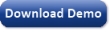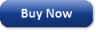Time Limited Trial

Released February 19, 2014

Last update: 5.0.3883New Entries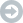Subscribe to the GEP-List

 3 8 4 0 1 2 3 4 5 6 7 8 9   0 1 2 3 4 5 6 7 8 9   0 1 2 3 4 5 6 7 8 9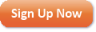Home | What's New | Products | Buy Now | Downloads | Quick Tour | Support | Contact Us | About Gepsoft | Sign UpForum | Blog | Videos | Tutorials | Server Knowledge Base | Logistic Regression Guide | Terms of Use | Privacy & Cookies